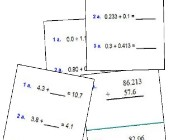Search

About 26 Search Results Matching Types of Worksheet, Worksheet Section, Generator, Generator Section, Subjects matching Math, Type matching Worksheet Section, Grades matching 3rd Grade, Similar to Math Worksheets for Kids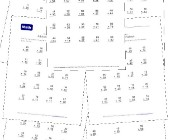Math Help - Addition Worksheets

This group of addition worksheets included a varie...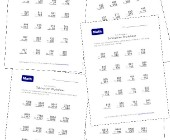Math Help Worksheets - Practice Subtraction

This group of subtraction worksheets includes a va...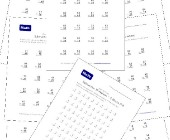Subtraction Worksheets for Kids

This set of free math worksheets is geared towards...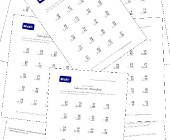Math Worksheets - Two Digit Subtraction

This group of subtraction worksheets is another gr...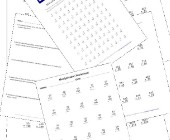Multiplication Worksheets for Kids

This set of math worksheets is geared towards help...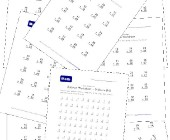This collection of addition worksheets includes si...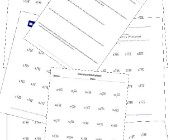Division Worksheets

Help kids learn division with this collection of m...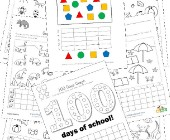Graphing Worksheets

Take a look at our collection of graphing workshee...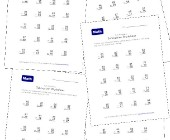Subtraction Worksheets - With Borrowing

This set of subtraction worksheets focuses on help...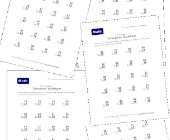Subtraction Worksheets - Without Borrowing

Check out this set of subtraction worksheets if yo...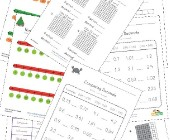Decimal Worksheets

This collection of free decimal worksheets will he...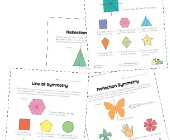Symmetry Worksheets

Help kids learn about the concept of symmetry with...